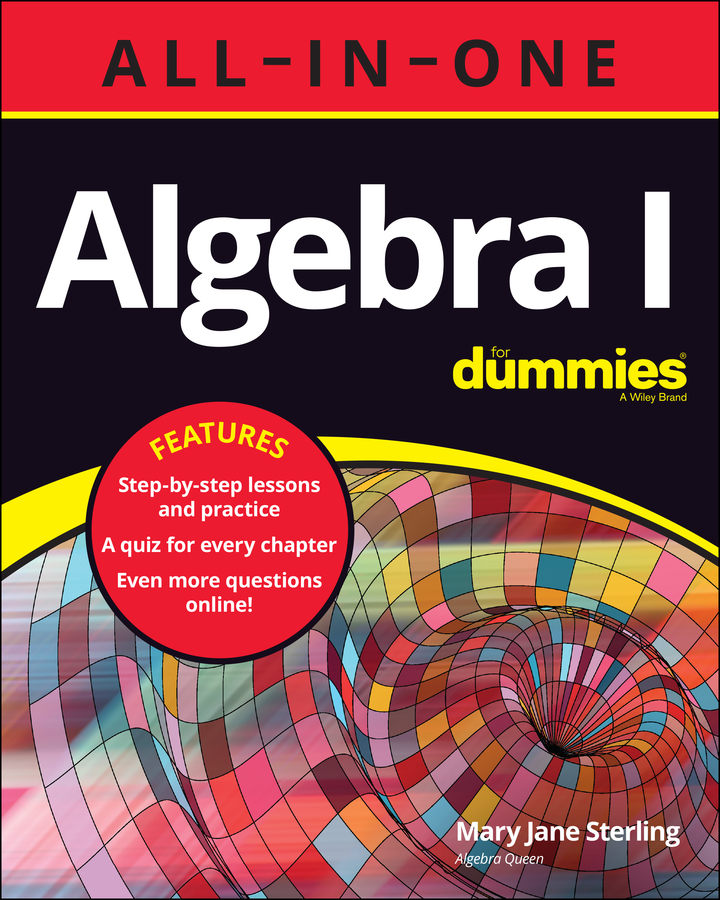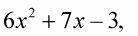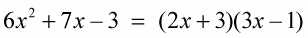##### Algebra I All-in-One For DummiesWhen you multiply two binomials, you can use the FOIL method. The letters in FOIL refer to two terms — one from each of two binomials — multiplied together in a certain order: First, Outer, Inner, and Last. Although the steps don't have to be done in this order, they usually are.are the result of multiplying two binomials together, so you can undo the multiplication by factoring them, which results in the two binomials (2x + 3)(3x – 1). But how do you know that it's factored correctly? By multiplying them using FOIL. Here's what the quadratic expression and its factored form looks like:The right side is the factored form. But how can you tell that the left side of that equation is equal to the right side just by looking at it? It’s not like a greatest common factor, where you look for something in common. Instead, you FOIL it:

F stands for the first term in each binomial: (3a + 6)(2a – 1)

O stands for the two outer terms — those farthest to the left and right: (3a + 6)(2a1)

I stands for the inner terms in the middle: (3a + 6)(2a – 1)

L stands for the last term in each binomial: (3a + 6)(2a1)

In each binomial, there’s the left term and the right term. But the two terms also have other names. The other names for the terms in the binomials refer to their positions in terms of the whole picture.

Example: (a + b)(c + d)

• The terms a and c are first.

• The terms a and d are outer.

• The terms b and c are inner.

• The terms b and d are last.